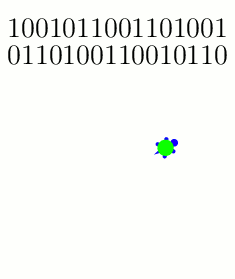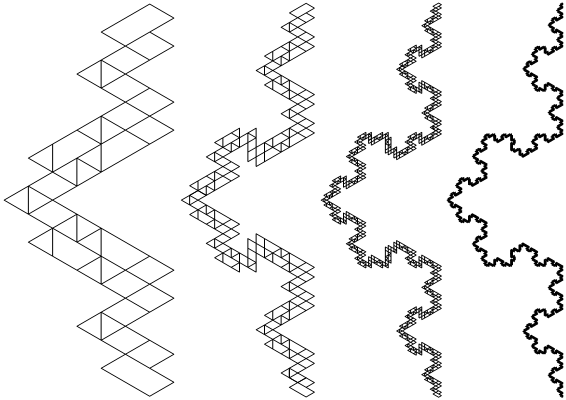# Thue-Morse Navigating Turtles

Here’s a simple rule to generate a pretty sequence of 1s and 0s: Start with the list 1 (just one digit) and repeatedly form a new list by flipping all the bits (1s become 0s and vice versa) and attaching this to the end: $$1 \to 10 \to 1001 \to 10010110 \to 1001011001101001 \to \cdots.$$ For example, to get from 1001 to the next sequence, we swap 1s and 0s to form 0110, and then we join these together to make 10010110. By continuing this process we can make our list longer and longer, and the limiting sequence $$1001011001101001011010011001011001101\cdots$$ is called the Thue-Morse Sequence.

What did I mean by calling this sequence “pretty”? Well, what does this sequence “look like”? One way to visualize it is with a turtle program. Imagine a turtle (let’s call him Leo) walking along the plane, and treat the digits of the Thue-Morse sequence as a list of instructions for the turtle as follows: When Leo sees the digit 1, he steps forward one foot; a 0 tells Leo to turn left by 60 degrees. What does Leo’s path look like? Here are the first 32 instructions he follows:The turtle follows these instructions on the first 32 entries of the Thue-Morse sequence: {1: Step; 0: Turn 60}.

And here are snapshots of his path as he gets farther into the Thue-Morse sequence:Snapshots of the turtle's trajectories after 2^8, 2^10, 2^12, and 2^14 instructions, respectively.

Why did we pick these instructions? What if Leo turns 120 degrees instead of 60 degrees when he sees a 0?The turtle follows the Thue-Morse sequence with a slightly different set of instructions: {1: Step; 0: Turn 120}. Shown here after 2^6, 2^8, 2^10, and 2^12 instructions, respectively.

If you zoom out and/or squint a little, these paths are roughly following a famous fractal called the Koch curve. And indeed, the more steps you draw, the closer the resemblance will become (as analyzed in these two papers: [MH2005] and [KZH2008]). But why stop here? What happens if we change the turtle’s instructions even more? I played around with some new rule sets, and these too show how the Koch curve seems innately “programmed” into the structure of the Thue-Morse sequence:Three more pretty examples of the Thue-Morse sequence's "turtle power." In all three cases, the trajectories converge to the Koch curve. Left: {1: (Turn 180, Step); 0: (Turn 60, Step)}, after 2^8 instructions. Middle: {1: (Turn 180, Step); 0: (Turn 120, Step)}, after 2^10 instructions. Right: {1: Turn 180; 0: (Turn 15, Step)}, after 2^10 instructions.

Here’s a final challenge: if the Koch curve and the Thue-Morse sequence are so intimately related, shouldn’t we be able to find some turtle program instructions that trace the Koch curve without “squinting”? Specifically, the Koch curve is usually constructed as the limit of the following sequence of paths, where each iteration is made by stitching together four copies of the previous curve:The Koch curve is constructed as the limit of these paths, where each curve is formed from four (shrunken) copies of the previous curve.

Is there a set of turtle instructions whose path traces exactly these curves? In fact, there is! These instructions work: {1: (Step, Turn 60); 0: Turn 180}.